# 24. Functions

## syntax

The concept of a function is one of the most important in mathematics. A common usage of functions in computer languages ​​is to implement mathematical functions. Such a function is computing one or more results, which are entirely determined by the parameters passed to it.

This is mathematics, but we are talking about programming and Python. So what is a function in programming? In the most general sense, a function is a structuring element in programming languages ​​to group a bunch of statements so they can be utilized in a program more than once. The only way to accomplish this without functions would be to reuse code by copying it and adapting it to different contexts, which would be a bad idea. Redundant code - repeating code in this case - should be avoided! Using functions usually enhances the comprehensibility and quality of a program. It also lowers the cost for development and maintenance of the software.

Functions are known under various names in programming languages, eg as subroutines, routines, procedures, methods, or subprograms.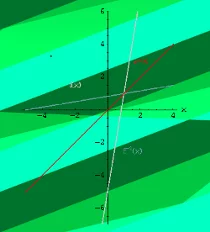Live Python training

Enjoying this page? We offer live Python training courses covering the content of this site.

Enrol here

## Motivating Example of Functions

Let's look at the following code:

print ( "Program starts" )

print ( "Hi Peter" )
print ( "Nice to see you again!" )
print ( "Enjoy our video!" )

# some lines of codes

print ( "Hi Sarah" )
print ( "Nice to see you again!" )
print ( "Enjoy our video!" )

width ,  length  =  3 ,  4
area  =  width  *  length

print("Hi Dominque")
print("Nice to see you again!")
print("Enjoy our video!")


#### OUTPUT:

Program starts
Hi Peter
Nice to see you again!
Enjoy our video!
Hi Sarah
Nice to see you again!
Enjoy our video!
Hi Dominque
Nice to see you again!
Enjoy our video!


Let us have a closer look at the code above: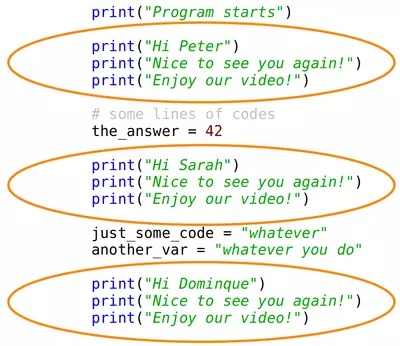You can see in the code that we are greeting three persons. Every time we use three print calls which are nearly the same. Just the name is different. This is what we call redundant code. We are repeating the code the times. This shouldn't be the case. This is the point where functions can and should be used in Python.

We could use wildcards in the code instead of names. In the following diagram we use a piece of the puzzle. We only write the code once and replace the puzzle piece with the corresponding name: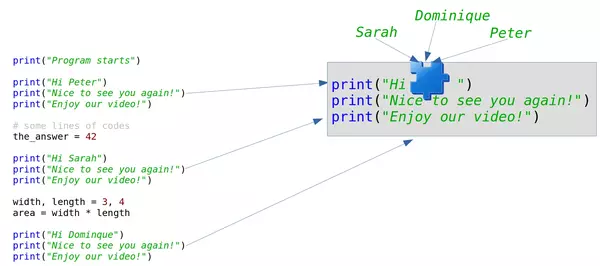Of course, this was no correct Python code. We show how to do this in Python in the following section.

## Functions in Python

The following code uses a function with the name greet. The previous puzzle piece is now a parameter with the name "name":

def greet(name):
print("Hi " + name)
print("Nice to see you again!")
print("Enjoy our video!")

print("Program starts")

greet("Peter")

# some lines of codes

greet("Sarah")

width, length = 3, 4
area = width * length

greet("Dominque")


#### OUTPUT:

Program starts
Hi Peter
Nice to see you again!
Enjoy our video!
Hi Sarah
Nice to see you again!
Enjoy our video!
Hi Dominque
Nice to see you again!
Enjoy our video!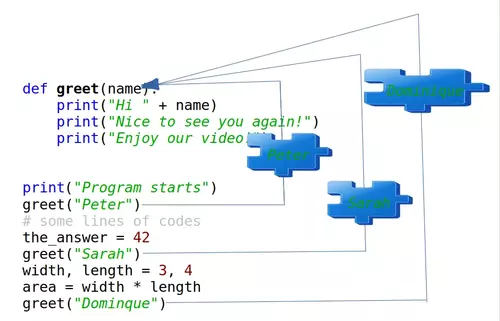We used a function in the previous code. We saw that a function definition starts with the def keyword. The general syntax looks like this:

def function-name(Parameter list):
statements, i.e. the function body

The parameter list consists of none or more parameters. Parameters are called arguments, if the function is called. The function body consists of indented statements. The function body gets executed every time the function is called. We demonstrate this in the following picture: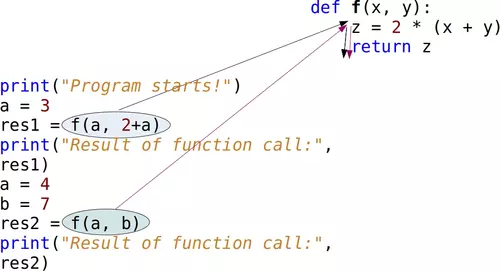The code from the picture can be seen in the following:

def f(x, y):
z = 2 * (x + y)
return z

print("Program starts!")
a = 3
res1 = f(a, 2+a)
print("Result of function call:", res1)
a = 4
b = 7
res2 = f(a, b)
print("Result of function call:", res2)


#### OUTPUT:

Program starts!
Result of function call: 16
Result of function call: 22


We call the function twice in the program. The function has two parameters, which are called x and y. This means that the function f is expecting two values, or I should say "two objects". Firstly, we call this function with f(a, 2+a). This means that a goes to x and the result of 2+a (5) 'goes to' the variable y. The mechanism for assigning arguments to parameters is called argument passing. When we reach the return statement, the object referenced by z will be return, which means that it will be assigned to the variable res1. After leaving the function f, the variable z and the parameters x and y will be deleted automatically.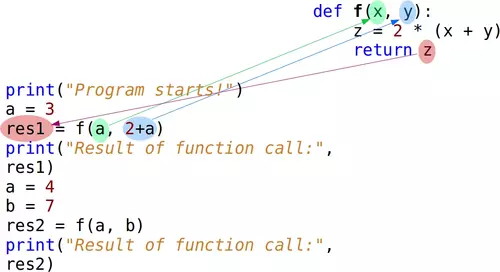The references to the objects can be seen in the next diagram: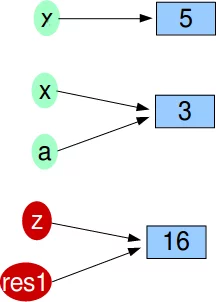The next Python code block contains an example of a function without a return statement. We use the pass statement inside of this function. pass is a null operation. This means that when it is executed, nothing happens. It is useful as a placeholder in situations when a statement is required syntactically, but no code needs to be executed:

def doNothing():
pass


A more useful function:

def fahrenheit(T_in_celsius):
""" returns the temperature in degrees Fahrenheit """
return (T_in_celsius * 9 / 5) + 32

for t in (22.6, 25.8, 27.3, 29.8):
print(t, ": ", fahrenheit(t))


#### OUTPUT:

22.6 :  72.68
25.8 :  78.44
27.3 :  81.14
29.8 :  85.64


""" returns the temperature in degrees Fahrenheit """ is the so-called docstring. It is used by the help function:

help(fahrenheit)


#### OUTPUT:

Help on function fahrenheit in module __main__:
fahrenheit(T_in_celsius)
returns the temperature in degrees FahrenheitOur next example could be interesting for the calorie-conscious Python learners. We also had an exercise in the chapter Conditional Statements of our Python tutorial. We will create now a function version of the program.

The body mass index (BMI) is a value derived from the mass (w) and height (l) of a person. The BMI is defined as the body mass divided by the square of the body height, and is universally expressed

$BMI = {w \over {l ^ 2}}$

The weight is given in kg and the length in metres.

def BMI(weight, height):
""" calculates the BMI where
weight is in kg and height in metres"""
return weight / height**2


We like to write a function to evaluate the bmi values according to the following table: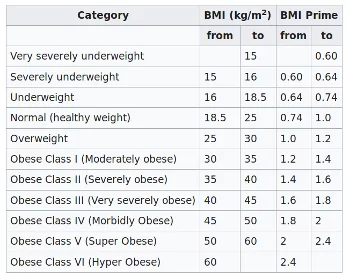The following shows code which is directly calculating the bmi and the evaluation of the bmi, but it is not using functions:

height = float(input("What is your height? "))
weight = float(input("What is your weight? "))

bmi = weight / height ** 2
print(bmi)
if bmi < 15:
print("Very severely underweight")
elif bmi < 16:
print("Severely underweight")
elif bmi < 18.5:
print("Underweight")
elif bmi < 25:
print("Normal (healthy weight)")
elif bmi < 30:
print("Overweight")
elif bmi < 35:
print("Obese Class I (Moderately obese)")
elif bmi < 40:
print("Obese Class II (Severely obese)")
else:
print("Obese Class III (Very severely obese)")


#### OUTPUT:

23.69576446280992
Normal (healthy weight)


Turn the previous code into proper functions and function calls.

def BMI(weight, height):
""" calculates the BMI where
weight is in kg and height in metres"""
return weight / height**2

def bmi_evaluate(bmi_value):
if bmi_value < 15:
result = "Very severely underweight"
elif bmi_value < 16:
result = "Severely underweight"
elif bmi_value < 18.5:
result = "Underweight"
elif bmi_value < 25:
result = "Normal (healthy weight)"
elif bmi_value < 30:
result = "Overweight"
elif bmi_value < 35:
result = "Obese Class I (Moderately obese)"
elif bmi_value < 40:
result = "Obese Class II (Severely obese)"
else:
result = "Obese Class III (Very severely obese)"
return result


Let us check these functions:

height = float(input("What is your height? "))
weight = float(input("What is your weight? "))

res = BMI(weight, height)
print(bmi_evaluate(res))


#### OUTPUT:

Normal (healthy weight)


Live Python training

Enjoying this page? We offer live Python training courses covering the content of this site.

Upcoming online Courses

Enrol here

## Default arguments in Python

When we define a Python function, we can set a default value to a parameter. If the function is called without the argument, this default value will be assigned to the parameter. This makes a parameter optional. To say it in other words: Default parameters are parameters, which don't have to be given, if the function is called. In this case, the default values are used.

We will demonstrate the operating principle of default parameters with a simple example. The following function hello, - which isn't very useful, - greets a person. If no name is given, it will greet everybody:

def hello(name="everybody"):
""" Greets a person """
result = "Hello " + name + "!")

hello("Peter")
hello()


#### OUTPUT:

Hello Peter!
Hello everybody!


## The Defaults Pitfall

In the previous section we learned about default parameters. Default parameters are quite simple, but quite often programmers new to Python encounter a horrible and completely unexpected surprise. This surprise arises from the way Python treats the default arguments and the effects steming from mutable objects.

Mutable objects are those which can be changed after creation. In Python, dictionaries are examples of mutable objects. Passing mutable lists or dictionaries as default arguments to a function can have unforeseen effects. Programmer who use lists or dictionaries as default arguments to a function, expect the program to create a new list or dictionary every time that the function is called. However, this is not what actually happens. Default values will not be created when a function is called. Default values are created exactly once, when the function is defined, i.e. at compile-time.

Let us look at the following Python function "spammer" which is capable of creating a "bag" full of spam:

def spammer(bag=[]):
bag.append("spam")
return bag


Calling this function once without an argument, returns the expected result:

spammer()


#### OUTPUT:

['spam']

The surprise shows when we call the function again without an argument:

spammer()


#### OUTPUT:

['spam', 'spam']

Most programmers will have expected the same result as in the first call, i.e. ['spam']

To understand what is going on, you have to know what happens when the function is defined. The compiler creates an attribute __defaults__:

def spammer(bag=[]):
bag.append("spam")
return bag

spammer.__defaults__


#### OUTPUT:

([],)

Whenever we will call the function, the parameter bag will be assigned to the list object referenced by spammer.__defaults__:

for i in range(5):
print(spammer())

print("spammer.__defaults__", spammer.__defaults__)


#### OUTPUT:

['spam']
['spam', 'spam']
['spam', 'spam', 'spam']
['spam', 'spam', 'spam', 'spam']
['spam', 'spam', 'spam', 'spam', 'spam']
spammer.__defaults__ (['spam', 'spam', 'spam', 'spam', 'spam'],)


Now, you know and understand what is going on, but you may ask yourself how to overcome this problem. The solution consists in using the immutable value None as the default. This way, the function can set bag dynamically (at run-time) to an empty list:

def spammer(bag=None):
if bag is None:
bag = []
bag.append("spam")
return bag

for i in range(5):
print(spammer())

print("spammer.__defaults__", spammer.__defaults__)


#### OUTPUT:

['spam']
['spam']
['spam']
['spam']
['spam']
spammer.__defaults__ (None,)


Live Python training

Enjoying this page? We offer live Python training courses covering the content of this site.

Enrol here

## Docstring

The first statement in the body of a function is usually a string statement called a Docstring, which can be accessed with the function_name.__doc__. For example:

def hello(name="everybody"):
""" Greets a person """
print("Hello " + name + "!")

print("The docstring of the function hello: " + hello.__doc__)


#### OUTPUT:

The docstring of the function hello:  Greets a person


## Keyword Parameters

Using keyword parameters is an alternative way to make function calls. The definition of the function doesn't change. An example:

def sumsub(a, b, c=0, d=0):
return a - b + c - d

print(sumsub(12, 4))
print(sumsub(42, 15, d=10))


#### OUTPUT:

8
17


Keyword parameters can only be those, which are not used as positional arguments. We can see the benefit in the example. If we hadn't had keyword parameters, the second call to function would have needed all four arguments, even though the c argument needs just the default value:

print(sumsub(42,15,0,10))


#### OUTPUT:

17


Live Python training

Enjoying this page? We offer live Python training courses covering the content of this site.

Enrol here

## Return Values

In our previous examples, we used a return statement in the function sumsub but not in Hello. So, we can see that it is not mandatory to have a return statement. But what will be returned, if we don't explicitly give a return statement. Let's see:

def no_return(x, y):
c = x + y

res = no_return(4, 5)
print(res)


#### OUTPUT:

None


If we start this little script, None will be printed, i.e. the special value None will be returned by a return-less function. None will also be returned, if we have just a return in a function without an expression:

def empty_return(x, y):
c = x + y
return

res = empty_return(4, 5)
print(res)


#### OUTPUT:

None


Otherwise the value of the expression following return will be returned. In the next example 9 will be printed:

def return_sum(x, y):
c = x + y
return c

res = return_sum(4, 5)
print(res)


#### OUTPUT:

9


Let's summarize this behavior: Function bodies can contain one or more return statements. They can be situated anywhere in the function body. A return statement ends the execution of the function call and "returns" the result, i.e. the value of the expression following the return keyword, to the caller. If the return statement is without an expression, the special value None is returned. If there is no return statement in the function code, the function ends, when the control flow reaches the end of the function body and the value None will be returned.

## Returning Multiple Values

A function can return exactly one value, or we should better say one object. An object can be a numerical value, like an integer or a float. But it can also be e.g. a list or a dictionary. So, if we have to return, for example, 3 integer values, we can return a list or a tuple with these three integer values. That is, we can indirectly return multiple values. The following example, which is calculating the Fibonacci boundary for a positive number, returns a 2-tuple. The first element is the Largest Fibonacci Number smaller than x and the second component is the Smallest Fibonacci Number larger than x. The return value is immediately stored via unpacking into the variables lub and sup:

def fib_interval(x):
""" returns the largest fibonacci
number smaller than x and the lowest
fibonacci number higher than x"""
if x < 0:
return -1
old, new = 0, 1
while True:
if new < x:
old, new = new, old+new
else:
if new == x:
new = old + new
return (old, new)

while True:
if x <= 0:
break
lub, sup = fib_interval(x)
print("Largest Fibonacci Number smaller than x: " + str(lub))
print("Smallest Fibonacci Number larger than x: " + str(sup))


#### OUTPUT:

Largest Fibonacci Number smaller than x: 5
Smallest Fibonacci Number larger than x: 8


Live Python training

Enjoying this page? We offer live Python training courses covering the content of this site.

Enrol here

## Local and Global Variables in Functions

Variable names are by default local to the function, in which they get defined.

def f():
print(s)      # free occurrence of s in f

s = "Python"
f()


#### OUTPUT:

Python

def f():
s = "Perl"     # now s is local in f
print(s)

s = "Python"
f()
print(s)


#### OUTPUT:

Perl
Python

def f():
print(s)        # This means a free occurrence, contradiction to bein local
s = "Perl"      # This makes s local in f
print(s)

s = "Python"
f()
print(s)


#### OUTPUT:

---------------------------------------------------------------------------
UnboundLocalError                         Traceback (most recent call last)
<ipython-input-25-81b2fbbc4d42> in <module>
6
7 s = "Python"
----> 8 f()
9 print(s)
<ipython-input-25-81b2fbbc4d42> in f()
1 def f():
----> 2     print(s)
3     s = "Perl"
4     print(s)
5
UnboundLocalError: local variable 's' referenced before assignment

If we execute the previous script, we get the error message: UnboundLocalError: local variable 's' referenced before assignment.

The variable s is ambigious in f(), i.e. in the first print in f() the global s could be used with the value "Python". After this we define a local variable s with the assignment s = "Perl".

def f():
global s
print(s)
s = "dog"
print(s)
s = "cat"
f()
print(s)


#### OUTPUT:

cat
dog
dog


We made the variable s global inside of the script. Therefore anything we do to s inside of the function body of f is done to the global variable s outside of f.

def f():
global s
print(s)
s = "dog"       # globally changed
print(s)

def g():
s = "snake"     # local s
print(s)

s = "cat"
f()
print(s)
g()
print(s)


#### OUTPUT:

cat
dog
dog
snake
dog


## Arbitrary Number of Parameters

There are many situations in programming, in which the exact number of necessary parameters cannot be determined a-priori. An arbitrary parameter number can be accomplished in Python with so-called tuple references. An asterisk "*" is used in front of the last parameter name to denote it as a tuple reference. This asterisk shouldn't be mistaken for the C syntax, where this notation is connected with pointers. Example:

def arithmetic_mean(first, *values):
""" This function calculates the arithmetic mean of a non-empty
arbitrary number of numerical values """

return (first + sum(values)) / (1 + len(values))

print(arithmetic_mean(45,32,89,78))
print(arithmetic_mean(8989.8,78787.78,3453,78778.73))
print(arithmetic_mean(45,32))
print(arithmetic_mean(45))


#### OUTPUT:

61.0
42502.3275
38.5
45.0


This is great, but we have still have one problem. You may have a list of numerical values. Like, for example,

x = [3, 5, 9]


You cannot call it with

 arithmetic_mean(x)

because "arithmetic_mean" can't cope with a list. Calling it with


arithmetic_mean(x, x, x)


#### OUTPUT:

5.666666666666667

is cumbersome and above all impossible inside of a program, because list can be of arbitrary length.

The solution is easy: The star operator. We add a star in front of the x, when we call the function.

 arithmetic_mean(*x)


#### OUTPUT:

5.666666666666667

This will "unpack" or singularize the list.

A practical example for zip and the star or asterisk operator: We have a list of 4, 2-tuple elements:

my_list = [('a', 232),
('b', 343),
('c', 543),
('d', 23)]


We want to turn this list into the following 2 element, 4-tuple list:

 [('a', 'b', 'c', 'd'),
(232, 343, 543, 23)] 

This can be done by using the *-operator and the zip function in the following way:

list(zip(*my_list))


#### OUTPUT:

[('a', 'b', 'c', 'd'), (232, 343, 543, 23)]

Live Python training

Enjoying this page? We offer live Python training courses covering the content of this site.

Enrol here

## Arbitrary Number of Keyword Parameters

In the previous chapter we demonstrated how to pass an arbitrary number of positional parameters to a function. It is also possible to pass an arbitrary number of keyword parameters to a function as a dictionary. To this purpose, we have to use the double asterisk "**"

def f(**kwargs):
print(kwargs)

f()


#### OUTPUT:

{}

f(de="German",en="English",fr="French")


#### OUTPUT:

{'de': 'German', 'en': 'English', 'fr': 'French'}


One use case is the following:

def f(a, b, x, y):
print(a, b, x, y)
d = {'a':'append', 'b':'block','x':'extract','y':'yes'}
f(**d)


#### OUTPUT:

append block extract yes


## Exercises with Functions

### Exercise 1

Rewrite the "dog age" exercise from chapter Conditional Statements as a function.

The rules are:

• A one-year-old dog is roughly equivalent to a 14-year-old human being
• A dog that is two years old corresponds in development to a 22 year old person.
• Each additional dog year is equivalent to five human years.

### Exercise 2

Write a function which takes a text and encrypts it with a Caesar cipher. This is one of the simplest and most commonly known encryption techniques. Each letter in the text is replaced by a letter some fixed number of positions further in the alphabet.

What about decrypting the coded text?

The Caesar cipher is a substitution cipher.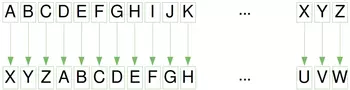### Exercise 3

We can create another substitution cipher by permutating the alphet and map the letters to the corresponding permutated alphabet.

Write a function which takes a text and a dictionary to decrypt or encrypt the given text with a permutated alphabet.

### Exercise 4

Write a function txt2morse, which translates a text to morse code, i.e. the function returns a string with the morse code.

Write another function morse2txt which translates a string in Morse code into a „normal“ string.

The Morse character are separated by spaces. Words by three spaces.### Exercise 5

Perhaps the first algorithm used for approximating $\sqrt{S}$ is known as the "Babylonian method", named after the Babylonians, or "Hero's method", named after the first-century Greek mathematician Hero of Alexandria who gave the first explicit description of the method.

If a number $x_n$ is close to the square root of $a$ then

$$x_{n+1} = \frac{1}{2}(x_n + \frac{a}{x_n})$$ will be a better approximation.

Write a program to calculate the square root of a number by using the Babylonian method.

### Exercise 6

Write a function which calculates the position of the n-th occurence of a string sub in another string s. If sub doesn't occur in s, -1 shall be returned.

### Exercise 7

Write a function fuzzy_time which expects a time string in the form hh: mm (e.g. "12:25", "04:56"). The function rounds up or down to a quarter of an hour. Examples:

fuzzy_time("12:58") ---> "13

Live Python training

Enjoying this page? We offer live Python training courses covering the content of this site.

Enrol here

## Solutions

### Solution to Exercise 1

def dog_age2human_age(dog_age):
"""dog_age2human_age(dog_age)"""
if dog_age == 1:
human_age = 14
elif dog_age == 2:
human_age = 22
else:
human_age =  22 + (dog_age-2)*5
return human_age

age = int(input("How old is your dog? "))
print(f"This corresponds to {dog_age2human_age(age)} human years!")


#### OUTPUT:

This corresponds to 37 human years!


### Solution to Exercise 2

import string
abc = string.ascii_uppercase
def caesar(txt, n, coded=False):
""" returns the coded or decoded text """
result = ""
for char in txt.upper():
if char not in abc:
result += char
elif coded:
result += abc[(abc.find(char) + n) % len(abc)]
else:
result += abc[(abc.find(char) - n) % len(abc)]
return result

n = 3
x = caesar("Hello, here I am!", n)
print(x)
print(caesar(x, n, True))


#### OUTPUT:

EBIIL, EBOB F XJ!
HELLO, HERE I AM!


In the previous solution we only replace the letters. Every special character is left untouched. The following solution adds some special characters which will be also permutated. Special charcters not in abc will be lost in this solution!

import string
abc = string.ascii_uppercase + " .,-?!"
def caesar(txt, n, coded=False):
""" returns the coded or decoded text """
result = ""
for char in txt.upper():
if coded:
result += abc[(abc.find(char) + n) % len(abc)]
else:
result += abc[(abc.find(char) - n) % len(abc)]
return result

n = 3
x = caesar("Hello, here I am!", n)
print(x)
print(caesar(x, n, True))

x = caesar("abcdefghijkl", n)
print(x)


#### OUTPUT:

EBIILZXEBOBXFX-J,
HELLO, HERE I AM!
-?!ABCDEFGHI


We will present another way to do it in the following implementation. The advantage is that there will be no calculations like in the previous versions. We do only lookups in the decrypted list 'abc_cipher':

import string

shift = 3
abc = string.ascii_uppercase + " .,-?!"
abc_cipher = abc[-shift:] + abc[:-shift]
print(abc_cipher)

def caesar (txt, shift):
"""Encodes the text "txt" to caesar by shifting it 'shift' positions """
new_txt=""
for char in txt.upper():
position = abc.find(char)
new_txt +=abc_cipher[position]
return new_txt

n = 3
x = caesar("Hello, here I am!", n)
print(x)

x = caesar("abcdefghijk", n)
print(x)


#### OUTPUT:

-?!ABCDEFGHIJKLMNOPQRSTUVWXYZ .,
EBIILZXEBOBXFX-J,
-?!ABCDEFGH


### Solution to Exercise 3

import string
from random import sample

alphabet = string.ascii_letters
permutated_alphabet = sample(alphabet, len(alphabet))

encrypt_dict = dict(zip(alphabet, permutated_alphabet))
decrypt_dict = dict(zip(permutated_alphabet, alphabet))

def encrypt(text, edict):
""" Every character of the text 'text'
is mapped to the value of edict. Characters
which are not keys of edict will not change"""
res = ""
for char in text:
res = res + edict.get(char, char)
return res

# Donald Trump: 5:19 PM, September 9 2014
txt = """Windmills are the greatest
threat in the US to both bald
and golden eagles. Media claims
fictional ‘global warming’ is worse."""

ctext = encrypt(txt, encrypt_dict)
print(ctext + "\n")
print(encrypt(ctext, decrypt_dict))


#### OUTPUT:

OQlerQGGk yDd xVd nDdyxdkx
xVDdyx Ql xVd Fz xo hoxV hyGe
yle noGedl dynGdk. gdeQy HGyQrk
EQHxQolyG ‘nGohyG MyDrQln’ Qk MoDkd.
Windmills are the greatest
threat in the US to both bald
and golden eagles. Media claims
fictional ‘global warming’ is worse.


Alternative solution:

import string
alphabet = string.ascii_lowercase + "äöüß .?!\n"

def caesar_code(alphabet, c, text, mode="encoding"):
text = text.lower()
coded_alphabet = alphabet[-c:] + alphabet[0:-c]
if mode == "encoding":
encoding_dict = dict(zip(alphabet, coded_alphabet))
elif mode == "decoding":
encoding_dict = dict(zip(coded_alphabet, alphabet))
print(encoding_dict)
result = ""
for char in text:
result += encoding_dict.get(char, "")
return result

txt2 = caesar_code(alphabet, 5, txt)
caesar_code(alphabet, 5, txt2, mode="decoding")


#### OUTPUT:

{'a': ' ', 'b': '.', 'c': '?', 'd': '!', 'e': '\n', 'f': 'a', 'g': 'b', 'h': 'c', 'i': 'd', 'j': 'e', 'k': 'f', 'l': 'g', 'm': 'h', 'n': 'i', 'o': 'j', 'p': 'k', 'q': 'l', 'r': 'm', 's': 'n', 't': 'o', 'u': 'p', 'v': 'q', 'w': 'r', 'x': 's', 'y': 't', 'z': 'u', 'ä': 'v', 'ö': 'w', 'ü': 'x', 'ß': 'y', ' ': 'z', '.': 'ä', '?': 'ö', '!': 'ü', '\n': 'ß'}
{' ': 'a', '.': 'b', '?': 'c', '!': 'd', '\n': 'e', 'a': 'f', 'b': 'g', 'c': 'h', 'd': 'i', 'e': 'j', 'f': 'k', 'g': 'l', 'h': 'm', 'i': 'n', 'j': 'o', 'k': 'p', 'l': 'q', 'm': 'r', 'n': 's', 'o': 't', 'p': 'u', 'q': 'v', 'r': 'w', 's': 'x', 't': 'y', 'u': 'z', 'v': 'ä', 'w': 'ö', 'x': 'ü', 'y': 'ß', 'z': ' ', 'ä': '.', 'ö': '?', 'ü': '!', 'ß': '\n'}
'windmills are the greatest \nthreat in the us to both bald \nand golden eagles. media claims \nfictional global warming is worse.'

### Solution to Exercise 4

latin2morse_dict = {'A':'.-', 'B':'-...', 'C':'-.-.', 'D':'-..',
'E':'.', 'F':'..-.', 'G':'--.','H':'....',
'I':'..', 'J':'.---', 'K':'-.-', 'L':'.-..',
'M':'--', 'N':'-.', 'O':'---', 'P':'.--.',
'Q':'--.-', 'R':'.-.', 'S':'...', 'T':'-',
'U':'..-', 'V':'...-', 'W':'.--', 'X':'-..-',
'Y':'-.--', 'Z':'--..', '1':'.----', '2':'...--',
'3':'...--', '4':'....-', '5':'.....', '6':'-....',
'7':'--...', '8':'---..', '9':'----.', '0':'-----',
',':'--..--', '.':'.-.-.-', '?':'..--..', ';':'-.-.-',
':':'---...', '/':'-..-.', '-':'-....-', '\'':'.----.',
'(':'-.--.-', ')':'-.--.-', '[':'-.--.-', ']':'-.--.-',
'{':'-.--.-', '}':'-.--.-', '_':'..--.-'}

# reversing the dictionary:
morse2latin_dict = dict(zip(latin2morse_dict.values(),
latin2morse_dict.keys()))

print(morse2latin_dict)


#### OUTPUT:

{'.-': 'A', '-...': 'B', '-.-.': 'C', '-..': 'D', '.': 'E', '..-.': 'F', '--.': 'G', '....': 'H', '..': 'I', '.---': 'J', '-.-': 'K', '.-..': 'L', '--': 'M', '-.': 'N', '---': 'O', '.--.': 'P', '--.-': 'Q', '.-.': 'R', '...': 'S', '-': 'T', '..-': 'U', '...-': 'V', '.--': 'W', '-..-': 'X', '-.--': 'Y', '--..': 'Z', '.----': '1', '...--': '3', '....-': '4', '.....': '5', '-....': '6', '--...': '7', '---..': '8', '----.': '9', '-----': '0', '--..--': ',', '.-.-.-': '.', '..--..': '?', '-.-.-': ';', '---...': ':', '-..-.': '/', '-....-': '-', '.----.': "'", '-.--.-': '}', '..--.-': '_'}

def txt2morse(txt, alphabet):
morse_code = ""
for char in txt.upper():
if char == " ":
morse_code += "   "
else:
morse_code += alphabet[char] + " "
return morse_code

def morse2txt(txt, alphabet):
res = ""
mwords = txt.split("   ")
for mword in mwords:
for mchar in mword.split():
res += alphabet[mchar]
res += " "
return res

mstring = txt2morse("So what?", latin2morse_dict)
print(mstring)
print(morse2txt(mstring, morse2latin_dict))


#### OUTPUT:

... ---    .-- .... .- - ..--..
SO WHAT?


### Solution to Exercise 5

def heron(a, eps=0.000000001):
""" Approximate the square root of a"""
previous = 0
new = 1
while abs(new - previous) > eps:
previous = new
new = (previous + a/previous) / 2
return new

print(heron(2))
print(heron(2, 0.001))


#### OUTPUT:

1.414213562373095
1.4142135623746899


### Solution to exercise 6

def findnth(s, sub, n):
num = 0
start = -1
while num < n:
start = s.find(sub, start+1)
if start == -1:
break
num += 1

return start

s = "abc xyz abc jkjkjk abc lkjkjlkj abc jlj"
print(findnth(s,"abc", 3))


#### OUTPUT:

19

def fuzzy_time(time):
hours, minutes = time.split(":")
minutes = int(minutes)
hours = int(hours)
minutes = minutes / 60   # Wandlung in Dezimalminutes
# Werte in 0.0, 0.25, 0.5, 0.75:
minutes = round(minutes * 4, 0) / 4
if minutes == 0:
print("About " + str(hours) + " o'clock!")
elif minutes == 0.25:
print("About a quarter past " + str(hours) + "!")
else:
if hours == 12:
hours = 1
else:
hours += 1
if minutes == 0.50:
print("About half past " + str ( hours )  +  "!" )
elif  minutes  ==  0.75 :
print ( "About a quarter to "  +  str ( hours )  +  "!" )
elif  minutes  ==  1.00 :
print ( "About "  +  str ( hours )  +  " o'clock!" )

hour  =  "12"
for  x  in  range ( 0 ,  60 ,  3 ):
fuzzy_time ( hour  + ":"  +  f " { x : 02d } " )


#### OUTPUT:

About 12 o'clock!